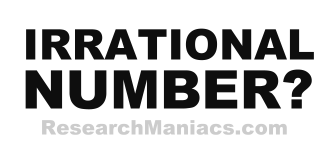Is 1 an Irrational Number?The number 1 is an irrational number if 1 canNOT be expressed as a ratio, as in irRATIOnal.

A quotient is the result you get when you divide one number by another number. For 1 to be an irrational number, the quotient of two integers canNOT equal 1.

In other words, for 1 to be an irrational number, 1 canNOT be expressed as a ratio where both the numerator and denominator are integers (whole numbers).

The number 1 can be expressed as 1/1 where 1 is the numerator and 1 is denominator.

Thus, the answer to the question "Is 1 an irrational number?" is NO.

Irrational Number?
Enter another number to see if it is irrational:

Is 2 an Irrational Number?
See information about the next number on our list.